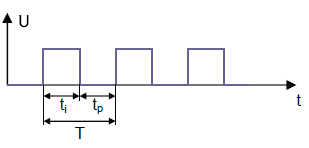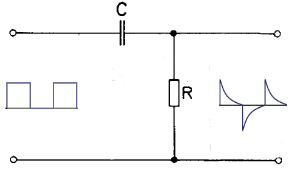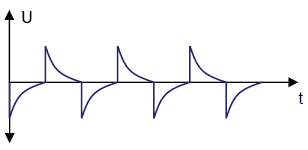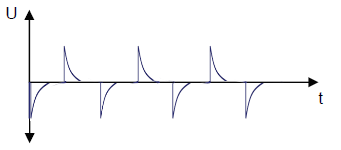# RC differentiator, calculate online

Calculator and formulas for calculating an RC differentiator

## Calculate RC differentiators online

With this function the properties of an RC differentiator can be calculated. The function calculates the capacitor, the resistance or the period or the frequency.

Calculate differentiators

 What should be calculated? Resistor Capacitor Frequency /Period Input Resistor mΩ Ω kΩ MΩ Capacitor F mF µF nF pF Frequency Period Pulse Hz kHz MHz GHz pSek nSek µSek mSek Sek pSek nSek µSek mSek Sek Decimal places 0 1 2 3 4 6 8 10 Result Resistor Capacitor Frequency Period

### Formulas for the RC differentiator

The differentiator works as a pulse shaper stage. The CR element generates a pulse-like alternating voltage at the output of the circuit from a square-wave voltage at the input.T = Period
t1 = PulseIf the length of the rectangular pulse (t1) corresponds to 5 times the time constant Τ of the CR element, a pulse train like the one in the figure below is created.$$\displaystyle t1= 5 · R · C$$   ⇒   $$\displaystyle R=\frac{t1}{5 · C}$$   ⇒   $$\displaystyle C=\frac{t1}{5 · R}$$

If the pulse duration of the input voltage is much greater than 5 T, short pulses equal to the input voltage are generated. The following figure shows a pulse train that occurs when the pulse length (t1) is 10 T.$$\displaystyle t1= 10 · R · C$$   ⇒   $$\displaystyle R=\frac{t1}{10 · C}$$   ⇒   $$\displaystyle C=\frac{t1}{10 · R}$$

### The time constant? (tau)

The time constant of an RC element is the product of R • C. The unit of measurement is the second. The symbol is the Greek letter $$τ$$ (tau),

After 5 tau the charge is approx. 99.3%.